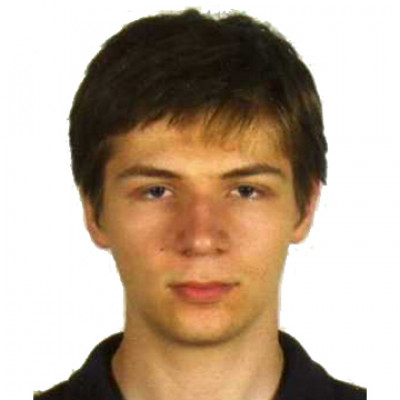# Peter Spacek

PhD student in Mathematics

`Peter's doctoral research, supervised by Dr Clelia Pech, is in the field of Mirror Symmetry, which is an idea originating from String Theory where two different models were found to be isomorphic for an appropriate choice of spaces. This idea has been brought over to abstract mathematics and translated to give a relation between symplectic structures on one space and complex structures on the other. His current research considers homogeneous spaces (which can be considered as quotients of Lie groups) and uses an algorithm to find the corresponding mirror spaces. This algorithm uses a combination of Algebraic Geometry and Lie Theory.   `
```Geometry in general: Differential, Symplectic, Complex and Riemannian Geometry.Theory behind the moduli space of stable curves and maps.Lie Theory in general.Derived categories, especially the derived category of coherent sheaves found in Mirror Symmetry.String Theory, especially the part that gave rise to the concept of Mirror Symmetry.Theory behind the moduli space of stable